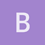Archive 04/01/2022.

[Query] Explain rate matrix in Phylogenetic analysisss107

The rate matrix (Q) for Jukes-Cantor generally is expressed as described here: https://en.wikipedia.org/wiki/Models_of_DNA_evolution#Most_common_models_of_DNA_evolution

The factor (1/4) comes because the base frequency is 0.25 in Jukes-Cantor analysis and μ is the rate of substitution.

The transition/probability matrix [P(t)] for any branch length (t) is the exponentiation of Q multiplied by t.

What I understand is: The matrix P will be entirely different if the substitution rate μ have two different values (say 0.25 and 1).

The question I have: Is there any restriction on the value of μ?

Related software packages like SeqGen/MrBayes expresses the rate matrix in terms of six rates {AC, AG, AT, CG, CT, GT} which all equals μ in this discussion.

From the examples from the related manuals, I think they normalize the rates such that their sum would be 1. That means, for JC, 6 μ = 1 always!

Generally, the substitution rates are taken as input either as percentages of the rate sum or they are scaled to the GT rate. I believe this information is not enough to decide the actual rate.

In simple terms, for JC, saying,

AC = AG = AT = CG = CT = GT

is not enough. You have to explicitly tell what the value is. Based on that value, the transition/probability matrix can change drastically.

If I always normalize the values then rate matrix for JC reduces to a matrix with static values.

I know some of my understanding is wrong. Where am I wrong?GrahamJonesGrahamJones

Ignore my last comment, I was trying to post from my new tablet.

I wanted to point you to this bit in the Wikipedia page:

It is worth noticing that v=(3/4)tu=…, [which is the] expected number of substitutions in time t (branch duration) for each particular site (per site) when the rate of substitution equals u.

Usually in phylogenetics software, v is normalised to 1.